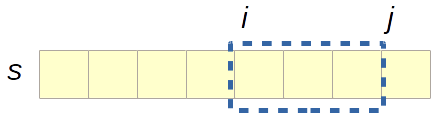# Programming-Idioms• Or search :

# Idiom #38 Extract a substring

Find substring t consisting in characters i (included) to j (excluded) of string s.
Character indices start at 0 unless specified otherwise.
Make sure that multibyte characters are properly handled.`T : String := S (I .. J - 1);`
```#include <stdlib.h>
#include <string.h>```
```char *t=malloc((j-i+1)*sizeof(char));
strncpy(t,s+i,j-i);```
`(def t (subs s i j))`
`#include <string>`
`auto t = s.substr(i, j-i);`
`var t = s.Substring(i, j - i);`
`auto t = s[i .. j];`
`var t = s.substring(i, j);`
`t = String.slice(s, i..j-1)`
`T = string:sub_string(I, J-1).`
```character(len=:), allocatable :: t

t = s(i:j-1)
```
`t := string([]rune(s)[i:j])`
`def t = s[i..<j]`
```t = drop i (take j s)
```
`let t = s.substring(i, j);`
`let t = s.slice(i, j);`
```String t = s.substring(i,j);
```
`val t = s.substring(i, j)`
```(setf u (subseq s i j))
```
`@import Foundation;`
`t=[s substringWithRange:NSMakeRange(i,j-i)]`
`\$t = mb_substr(\$s, \$i, \$j-\$i, 'UTF-8');`
`t := copy(s,i+1,j-i);`
`uses Sysutils;`
`t := s.Substring(i,j-i);`
`my \$chunk = substr("now is the time", \$i, \$j);`
`t = s[i:j]`
`t = s[i..j-1] `
```extern crate unicode_segmentation;
use unicode_segmentation::UnicodeSegmentation;```
`let t = s.graphemes(true).skip(i).take(j - i).collect::<String>();`
`use substring::Substring;`
`let t = s.substring(i, j);`
`(define t (substring s i j))`
`t := s copyFrom: i to: j - 1.`
`Dim t As String = s.Substring(i,j)`

programming-idioms.org